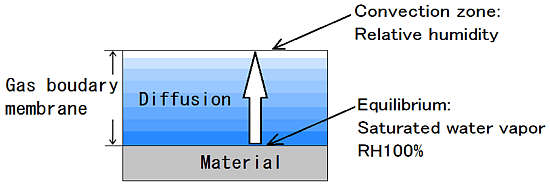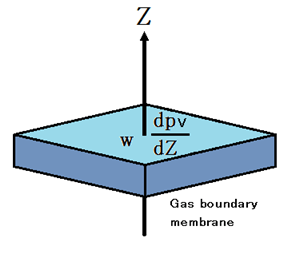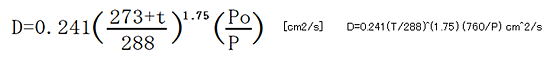# 3-2.Evaporation of waterIn order to move water vapor becomes water evaporates, the water vapor pressure difference is required between the air and the convection layer and the surface of the material.

Water vapor of the material surface so that the saturated pressure is the saturated water vapor pressure.
Pressure in the convection zone is proportional to the humidity.

As an example, (concentration 1.28mol/m3), relative humidity 70RH% will give the (0.896mol/m3 concentration) 2.22kPa 3.17kPa saturated water vapor pressure of 25 ℃.
3.17-2.22 = 0.95kPa of this pressure difference is the driving force of the diffusion of water vapor.

Take for example,
“es” is the saturated water vapor pressure of the material surface.
“e” is the water vapor pressure of the convective zone.
When es>e, evaporation proceeds water vapor moves to the convection zone.
When es<e, water vapor moves to the gas boundary layer from convection zone, or water vapor to condense on the surface, it is absorbed by the material.
It is called dew condensation that vapor condenses and liquefies on the surface.

Gas boundary membrane is produced by diffusion of water vapor in the material surface.
It depends on the state of the air flow, area of ​​the surface, and shape, the thickness of the Gas boundary membrane is the 1mm ~ 10mm or more.
Difference in the thickness of the gGas boundary membrane can affect the rate of evaporation of water.

The difference of density is born into the Gas boundary membrane, and water vapor will move in accordance with the gradient of the density.

Constant drying phase of the drying process appears the law of diffusion Fick in gas boundary membrane.w = -D dpv/dZ

w :Mass of water vapor that passes through the unit time
D :Diffusion coefficient
pv:Density of water vapor
dpv/dZ: Density gradient of water vapor in the Z-axis direction

(-) Sign, means that the water vapor moves in the direction in the density decreases.

The formula of the vapor diffusion coefficient Dt :Temperature ℃
Po:Standard atmospheric pressure (= 1013.25hPa)
P :The pressure of the air

The diffusion coefficient is proportional to the 1.75th power of the absolute temperature, and inversely proportional to pressure.

The diffusion coefficient of water vapor in standard atmospheric pressure is 0.220 cm [/s ]2 (0℃).

2.56 × 10-5m2 / s
It is known to be DAB = 2.88 × 10-5 m2 / s.

If this formula treats the air containing vapor as ideal gas, since the density of vapor is proportional to water vapor pressure, it can also use a vapor slope instead of a density gradient.

w = -DMv/RT x de/dZ

w :Mass of water vapor that passes through the unit time
D :Diffusion coefficient
Mv:Molar mass of water vapor
R :Universal gas constant
T :Absolute temperature
e :Partial pressure of water vapor density